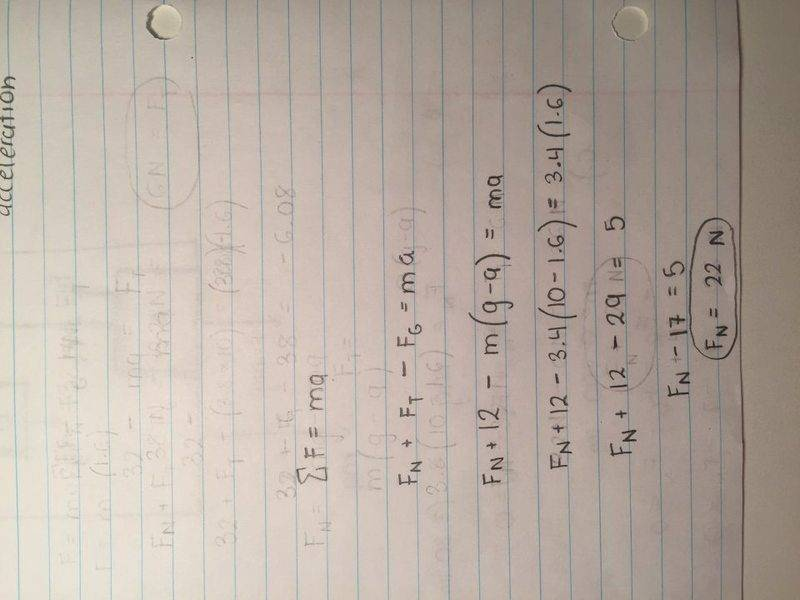# Forces acting on a box-pulley system in an elevator?

## Homework Statement

[/B]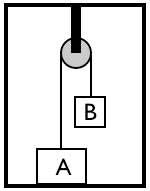For this problem, use
g = 10 N/kg.

As shown in the figure above, a pulley is mounted to the ceiling of an elevator. The elevator is initially traveling with a constant velocity of 1.40 m/s, directed up. The rope (which we assume to have no mass) passing over the pulley has block A tied to one end and block B tied to the other. The mass of block A is 3.80 kg. Block A remains on the floor of the elevator, at rest with respect to the elevator. The mass of block B is 1.40 kg, and block B is also at rest with respect to the elevator, hanging from the rope.

(a) Calculate the magnitude of the tension in the rope.
This is the only part of the problem I got correct. My answer: 14 N

I used the equation ...

(-1.4)(10) = -T
14 N = T

to find the tension in the rope attached to weight B, since I figured that only this block would be giving the rope a tension.

(b) Calculate the magnitude of the force exerted on block A by the floor of the elevator.

(c) The total mass of the pulley and its support is 0.700 kg. Calculate the magnitude of the force exerted by the ceiling of the elevator on the pulley support.

At some later time, the elevator is moving, and it has an acceleration of 1.60 m/s2, directed down. The blocks remain at rest with respect to the elevator.

(d) Calculate the magnitude of the tension in the rope now.

(e) Calculate the magnitude of the force exerted on block A by the floor of the elevator now.

I'm having difficulty solving for remaining portions of this problem. Please provide guidelines and an explanation for how I can go about answering the different parts of this question; I would really like to understand.

Thank you!

[/B]
ma - mg = -T

## The Attempt at a Solution

[/B]
Part a.)

I used the equation ...

(-1.4)(10) = -T
14 N = T

to find the tension in the rope attached to weight B, since I figured that only this block would be giving the rope a tension.

gneill
Mentor
Hello Sagrebella, Welcome to Physics Forums.

You'll need to show some attempt for each part of the problem that you need help on. As a general hint, always start by drawing a Free Body Diagram (FBD) for the components of the system that you are examining. For part (b), for example, draw the FBD for block A.

But I can't solve the other parts, I don't know how; this is why I am posting my question on this forum. Please provide guidelines for the individual parts and i'll show you my work once I receive them.

that is, once I receive guidelines ...

Hello Sagrebella, Welcome to Physics Forums.

You'll need to show some attempt for each part of the problem that you need help on. As a general hint, always start by drawing a Free Body Diagram (FBD) for the components of the system that you are examining. For part (b), for example, draw the FBD for block A.

But I can't solve the other parts, I don't know how; this is why I am posting my question on this forum. Please provide guidelines for the individual parts and then i'll show you my work ...

gneill
Mentor
But I can't solve the other parts, I don't know how; this is why I am posting my question on this forum. Please provide guidelines for the individual parts and then i'll show you my work ...
Alas, that's not how things work here; You need to show some attempt before help can be provided. I know it can be frustrating if you are a beginner and having difficulty with the basics, but somewhere in your course materials, notes or textbook, there must be a similar or related example to provide some hint of where to start. Teachers generally don't assign problems without first covering the necessary concepts. As I mentioned previously, drawing the FBD is always a good place to start. If you don't know what an FBD is, then you'll need to go back to your notes and text to find out.

Alas, that's not how things work here; You need to show some attempt before help can be provided. I know it can be frustrating if you are a beginner and having difficulty with the basics, but somewhere in your course materials, notes or textbook, there must be a similar or related example to provide some hint of where to start. Teachers generally don't assign problems without first covering the necessary concepts. As I mentioned previously, drawing the FBD is always a good place to start. If you don't know what an FBD is, then you'll need to go back to your notes and text to find out.

Ok, here is one of my best attempts. I've been working on this problem many times so far. I am really confused how to solve c.), so I left it blank. Could you please give me guidelines now? I would really like to understand this problem, I'm really not trying to get an easy homework pass.

gneill
Mentor
FYI, you can upload more than one image per post, and you can insert them in-line in your post text to make them full size by selecting the FULL IMAGE icon next to the thumbnail when you are creating your post.

It's unfortunate that your images are not oriented properly, but at least your text is clear. The formulas are simple enough that you would probably be better off typing them in so that they are easily quoted and commented on.

That said, for part (c) you defined a force Fp as "force of pulley". Can you elaborate on what that is?

That said, for part (c) you defined a force Fp as "force of pulley". Can you elaborate on what that is?

Sorry, "Force pulley" was a mistake. I erased it from the FBD and from the equation.

Anyways, can we solve the problem now? For the parts I did solve, are the answers correct or close to being correct?

Also, sorry, but I was unable to upload a "portrait oriented" image.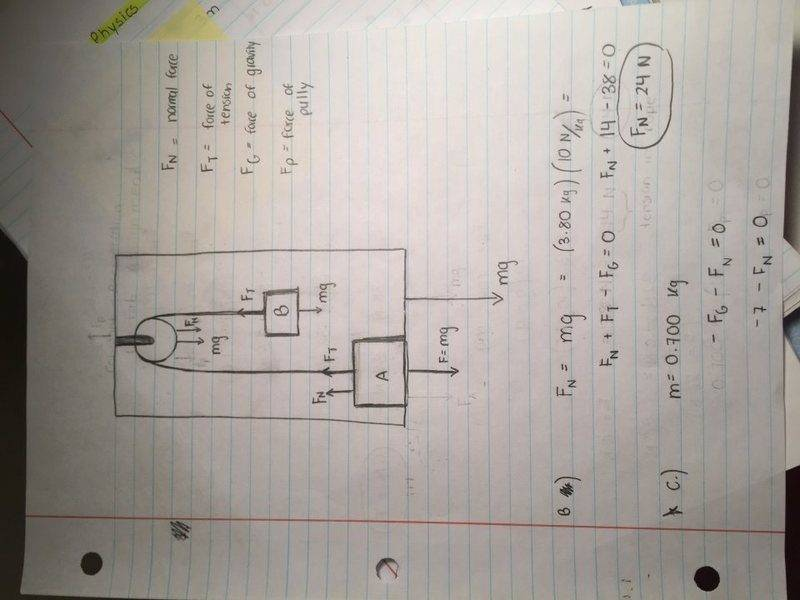gneill
Mentor

For part (c), what effect do you think the rope has on the pulley? (I was hoping that the Fp would represent that contribution). What force(s) are transmitted to the pulley by the rope?

Ah, ok, I see what you mean. Perhaps that's what I was originally thinking when I included Fp on my FBD, but after I returned to the problem, I forgot why I put it there in the first place.

Ok, so I re-did my work for part c.), taking into mind the contribution that the rope would transmit to the pulley. Would it be correct to say that Fp = Ft ?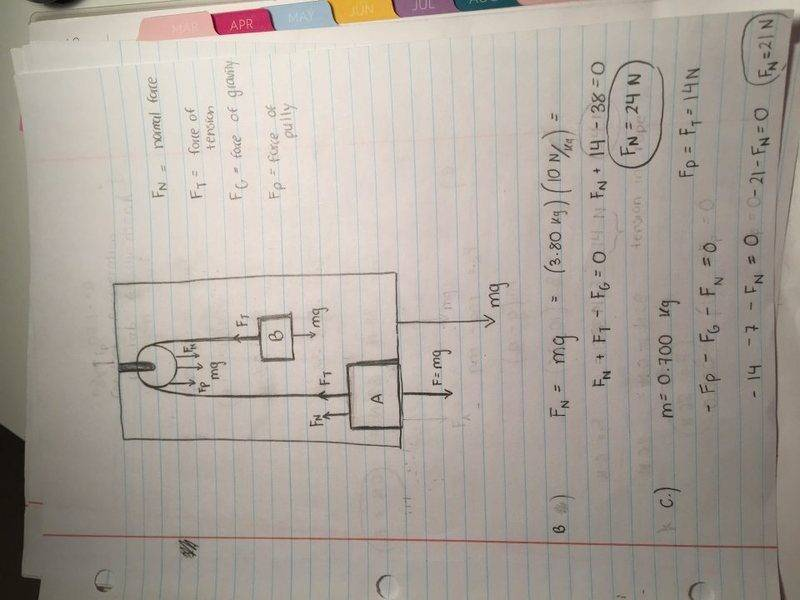gneill
Mentor
Ok, so I re-did my work for part c.), taking into mind the contribution that the rope would transmit to the pulley. Would it be correct to say that Fp = Ft ?
Close. Note that the rope "attaches" to the pulley twice. The tension is the same for both sides.

Ok, that makes sense.

I would multiply the Ft by two in order to account for the tension coming from both sides of the weight, correct?

So, in that case ...

Fp = Ft = 14N * 2 = 28N

which gives ...

-28 - 7 - Fn = 0
-35 - Fn = 0
Fn = 35N (directed down)

Close. Note that the rope "attaches" to the pulley twice. The tension is the same for both sides.

would you mind guiding me through d.) and e.) too please?

gneill
Mentor
Ok, that makes sense.

I would multiply the Ft by two in order to account for the tension coming from both sides of the weight, correct?

So, in that case ...

Fp = Ft = 14N * 2 = 28N

which gives ...

-28 - 7 - Fn = 0
-35 - Fn = 0
Fn = 35N (directed down)
That's right, but you might want to choose another variable name other than Fn since you used that name previously to mean the force exerted by the floor of the elevator on block A.

gneill
Mentor
For part (d), in your image for your second page of work (post #9) you showed the following: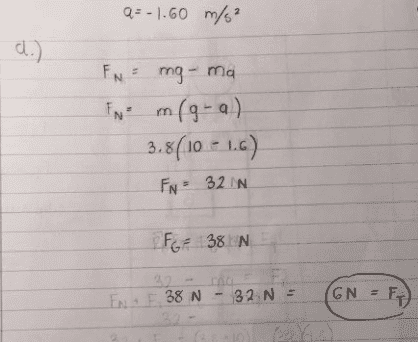What you have calculated here appears to be the change in weight for block A due to the accelerated motion of the elevator rather than the tension in the rope.

Compare this calculation with the method you used for part (a), which also asked for the tension in the rope: Shouldn't mass B play the same role here that it did there?

Ok, so I re-did my calculations with Block B in mind and got the tension of the rope to be 16 N

m(a-g) = - FtB

1.40(-1.6-10) = - FtB

16N = FtB

is this correct? would the overall tension be coming just from block B?

gneill
Mentor
Ok, so I re-did my calculations with Block B in mind and got the tension of the rope to be 16 N

m(a-g) = - FtB

1.40(-1.6-10) = - FtB

16N = FtB

is this correct? would the overall tension be coming just from block B?
You're on the right track but you need to be careful with the signs of the accelerations. The elevator is physically accelerating downwards so it "cancels" some of the effects of the acceleration due to gravity. Imagine for a moment that the elevator were accelerating downwards at g (such as if its lifting cable snapped and it went into free-fall). What would be the apparent weight of block B?

Now in this case the acceleration a is smaller than g but it is still directed downwards. So do you expect the tension in the rope to be larger or smaller than what you calculated in part (a)?

Would the elevator's downward acceleration be negative relative to g, since you mentioned that it "cancels" some of the effects of g?

making the equation

1.4(10-1.6) = Ft

Ft= 12N

Sorry, I'm still a little confused on this part .... so, if the cable were to snap, with the elevator reaching a downward acceleration of g, wouldn't the apparent weight of block B be smaller than it was at acceleration a?

And since the acceleration of Block B was 0 in part A, but is now 1.60 m/s2 in part D (an increase), would the tension in part B be larger than the tension in Part D?

Again, sorry if what I inferred is a bunch of nonsense. I'm still a little shaky with these concepts.

gneill
Mentor
Would the elevator's downward acceleration be negative relative to g, since you mentioned that it "cancels" some of the effects of g?
Yes. It's a effect of inertial mass. With downward acceleration of the elevator and its contents, their masses "resist" the acceleration (F = MA).
making the equation

1.4(10-1.6) = Ft

Ft= 12N
Yes, that looks good.
Sorry, I'm still a little confused on this part .... so, if the cable were to snap, with the elevator reaching a downward acceleration of g, wouldn't the apparent weight of block B be smaller than it was at acceleration a?
Yes, in fact in the frame of reference of the elevator, block B (and block A for that matter) would appear "weightless".
And since the acceleration of Block B was 0 in part A, but is now 1.60 m/s2 in part D (an increase), would the tension in part B be larger than the tension in Part D?
No, see above. If the elevator were accelerating upwards then the inertia of the masses would increase the apparent weights, effectively adding to the g. When you are in a car that's accelerating forwards, are you pressed back into your seat or pulled forward into the windscreen? That's an effect of inertia, your body mass resisting the accelerationAgain, sorry if what I inferred is a bunch of nonsense. I'm still a little shaky with these concepts.
No worries, everyone goes through the same learning experience when the concepts are new. Soon you'll wonder why it ever seemed so tricky.Yes. It's a effect of inertial mass. With downward acceleration of the elevator and its contents, their masses "resist" the acceleration (F = MA).

Yes, that looks good.

Yes, in fact in the frame of reference of the elevator, block B (and block A for that matter) would appear "weightless".

No, see above. If the elevator were accelerating upwards then the inertia of the masses would increase the apparent weights, effectively adding to the g. When you are in a car that's accelerating forwards, are you pressed back into your seat or pulled forward into the windscreen? That's an effect of inertia, your body mass resisting the accelerationNo worries, everyone goes through the same learning experience when the concepts are new. Soon you'll wonder why it ever seemed so tricky.Ah, ok, I think (hopefully) I understand better now: the effect of inertia is what acts on the masses and tension. The greater the upward acceleration, the greater the inertia of the masses (resistance to the acceleration), and the greater the tension in the rope (since the apparent weight of the masses increases and the rope needs to exert a greater upward "pull").

Ok, final part of the question: e.). Would you mind helping me with this last one too please? Your explanations are very helpfulgneill
Mentor
For part (e), apply the same method that you did in part (b), keeping in mind the new effective value of "g" that the masses experience.

Ok, here's my attempt, hopefully it's correct, or at least close to being correct. I will upload my work as a picture this time ...